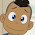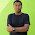## Wednesday, December 23, 2009

### VHDL code for Full Adder using structural style

library IEEE;
use IEEE.std_logic_1164.all;

entity bejoy_fa is
port(In1,In2,c_in : in std_logic;
sum, c_out : out std_logic);
end bejoy_fa;

architecture arc of bejoy_fa is

port(a,b : in std_logic;
sum, carry : out std_logic);
end component;

component or_2
port(a,b : in std_logic;
c : out std_logic);
end component;

signal s1, s2, s3 : std_logic;

begin

H1: half_adder port map(a=>In1, b=>In2, sum=>s1, carry=>s3);

H2: half_adder port map(a=>s1, b=>c_in, sum=>sum, carry=>s2);

O1: or_2 port map(a=> s2, b=>s3, c=>c_out);

end arc;

port (a,b : in bit ;
sum,carry : out bit);

begin

sum<= a xor b;
carry <= a and b;

end arc;

entity or_2 is

port (a,b : in bit ;
c : out bit);

end or_2;

architecture arc of or_2 is

begin

c<= a or b;

end arc;

1.thanks bejoy ! you were very helpful indeed.
thanks again buddy

2.iwant to become Ur friend

3.thank u

4.written in very easy language...understood very well.....thnks

5.if the half adder vhdl code is designed in structural style(using ex-or and and gate)then how to implement full adder in structural.

6.Great help indeed! Thank you! :)

7.thank you

8.super cool...

9.pls give the logical diagram of this code then it would be great

10.pls give the logical diagram of the code then it would be great

11.